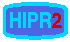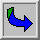# Image Arithmetic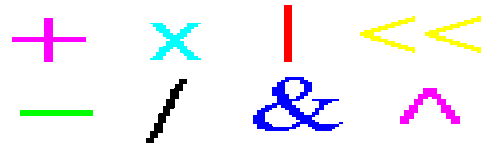# ContentsAddition - pointwise addition: image + image (or constant)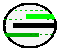Subtraction - pointwise subtraction: image - image (or constant)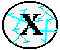Multiplication - pointwise multiplication: images * image (or constant)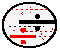Division - pointwise division: images / image (or constant)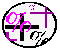Blending - pointwise linear combination of two images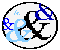Logical AND/NAND - pointwise logical ANDing/NANDing of two binary images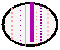Logical OR/NOR - pointwise logical ORing/NORing of two binary images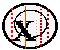Logical XOR/XNOR - pointwise logical XORing/XNORing of two binary imagesInvert/Logical NOT - pointwise inversion of a binary image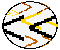Bitshift Operators - pointwise scaling of an imageImage arithmetic applies one of the standard arithmetic operations or a logical operator to two or more images. The operators are applied in a pixel-by-pixel fashion which means that the value of a pixel in the output image depends only on the values of the corresponding pixels in the input images. Hence, the images normally have to be of the same size. One of the input images may be a constant value, for example when adding a constant offset to an image.

Although image arithmetic is the most simple form of image processing, there is a wide range of applications. A main advantage of arithmetic operators is that the process is very simple and therefore fast.

In many applications the processed images are taken from the same scene at different points of time, as, for example, in reduction of random noise by adding successive images of the same scene or motion detection by subtracting two successive images.

Logical operators are often used to combine two (mostly binary) images. In the case of integer images, the logical operator is normally applied in a bitwise fashion. Then we can, for example, use a binary mask to select a particular region of an image.